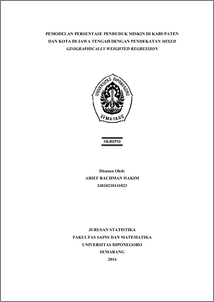# PEMODELAN PERSENTASE PENDUDUK MISKIN DI KABUPATEN DAN KOTA DI JAWA TENGAH DENGAN PENDEKATAN MIXED GEOGRAPHICALLY WEIGHTED REGRESSION

Hakim, Arief Rachman (2014) PEMODELAN PERSENTASE PENDUDUK MISKIN DI KABUPATEN DAN KOTA DI JAWA TENGAH DENGAN PENDEKATAN MIXED GEOGRAPHICALLY WEIGHTED REGRESSION. Undergraduate thesis, FSM Undip.Preview
PDF
724Kb

## Abstract

Regression analysis is a statistical analysis that models the relationship between the response variable and the predictor variable. Geographically Weighted Regression (GWR) is the development of linear regression with the added factor of the geographical location where the response variable is taken, so that the resulting parameters will be local. Mixed Geographically Weighted Regression (MGWR) has a basic concept that is a combination of a linear regression model and GWR, by modeling variables that are local and which are global variables. Methods for estimating the model parameters MGWR no different from the GWR using Weighted Least Square (WLS). Selection of the optimum bandwidth using the Cross Validation (CV). Application models MGWR the percentage of poor people in the district and town in Central Java showed MGWR models that different significantly from the global regression model. As well as models generated for each area will be different from each other. Based on the Akaike Information Criterion (AIC) between the global regression model, the GWR and MGWR models, it is known that MGWR models with Gaussian kernel weighting function is the best model is used to analyze the percentage of poor in the counties and cities in Central Java because it has the smallest AIC value . Keywords: Akaike Information Criterion, Cross Validation, Fungsi Kernel Gaussian, Mixed Geographically Weighted Regression, Weighted Least Square.

Item Type: Thesis (Undergraduate) H Social Sciences > HA Statistics Faculty of Science and Mathematics > Department of Statistics 46319 Mr Hasbi Yasin 02 Sep 2015 17:13 02 Sep 2015 17:13

Repository Staff Only: item control page